Miscellaneous

Chapter 11 Class 12 Three Dimensional Geometry
Serial order wise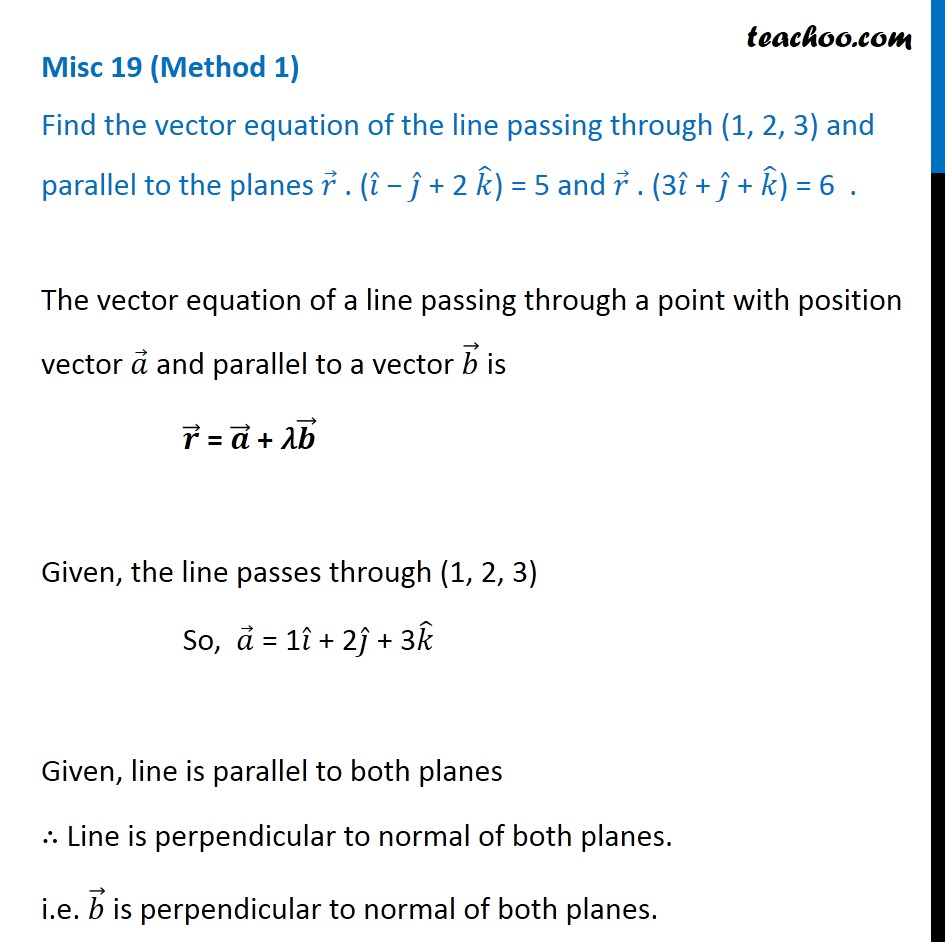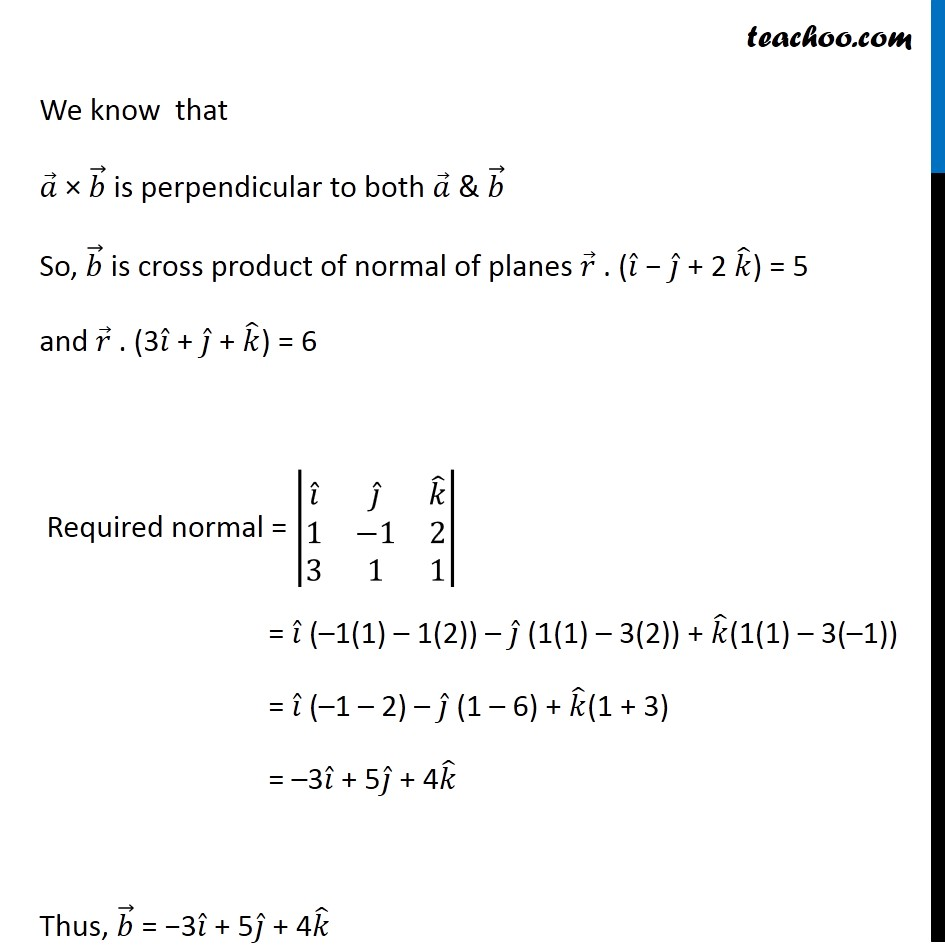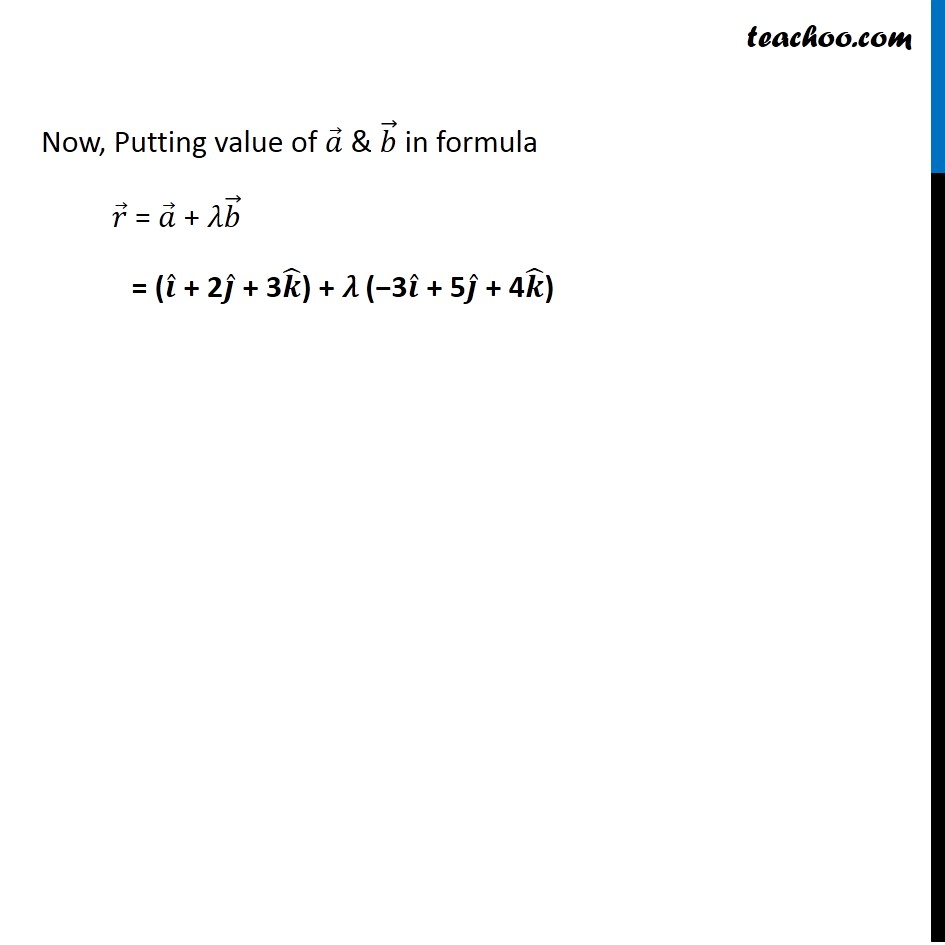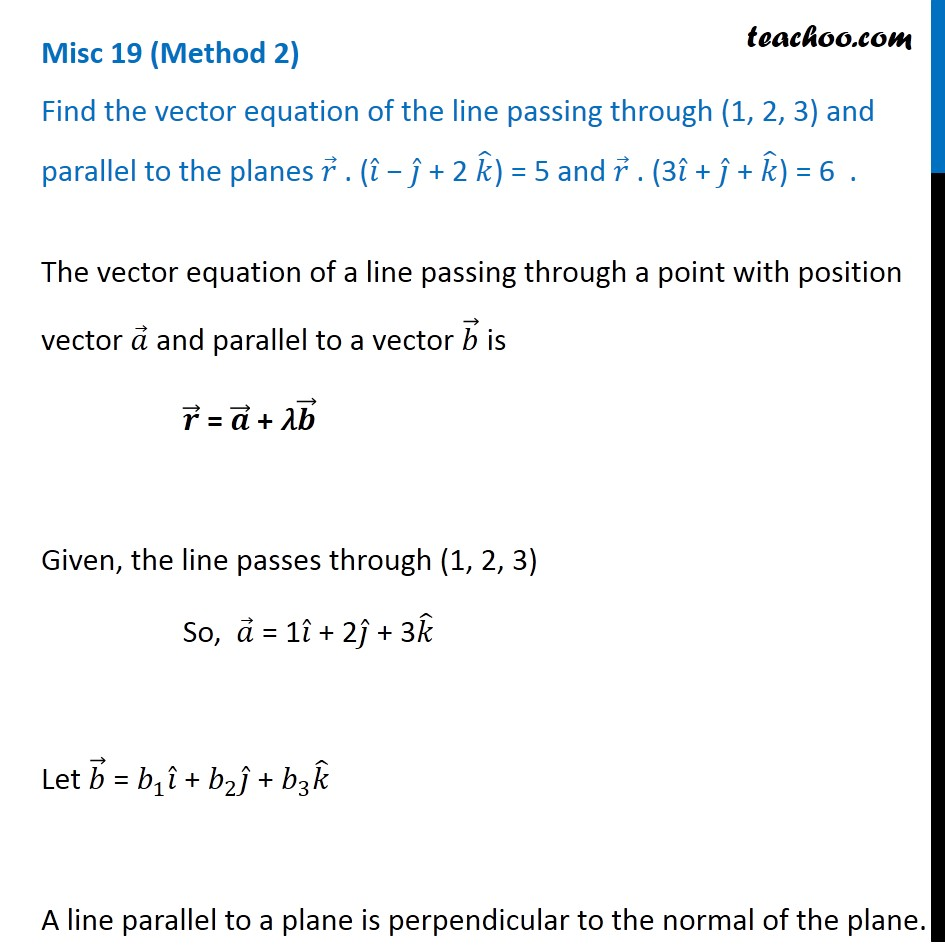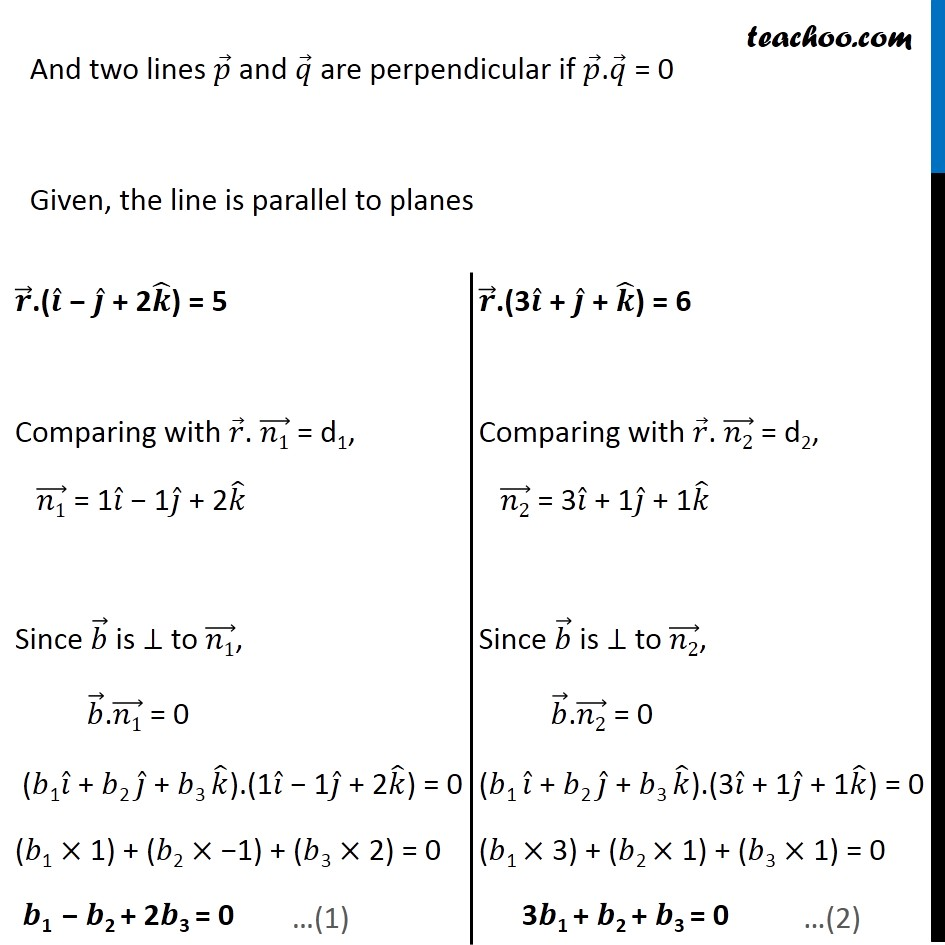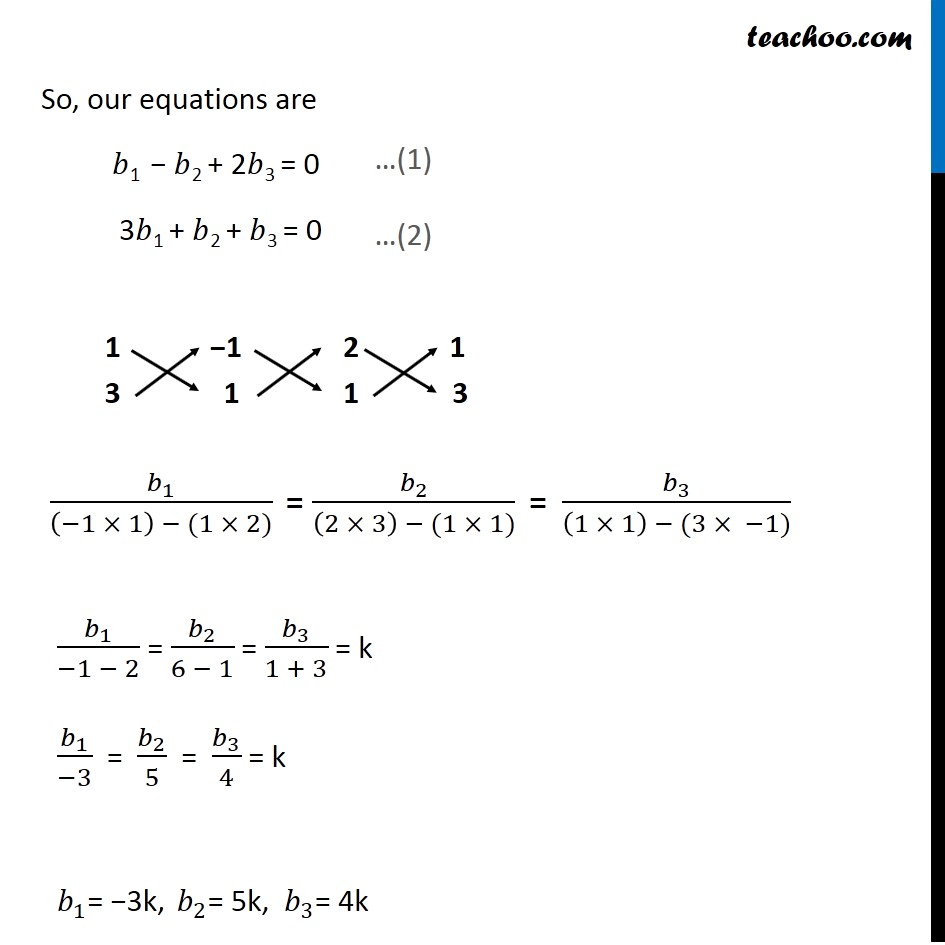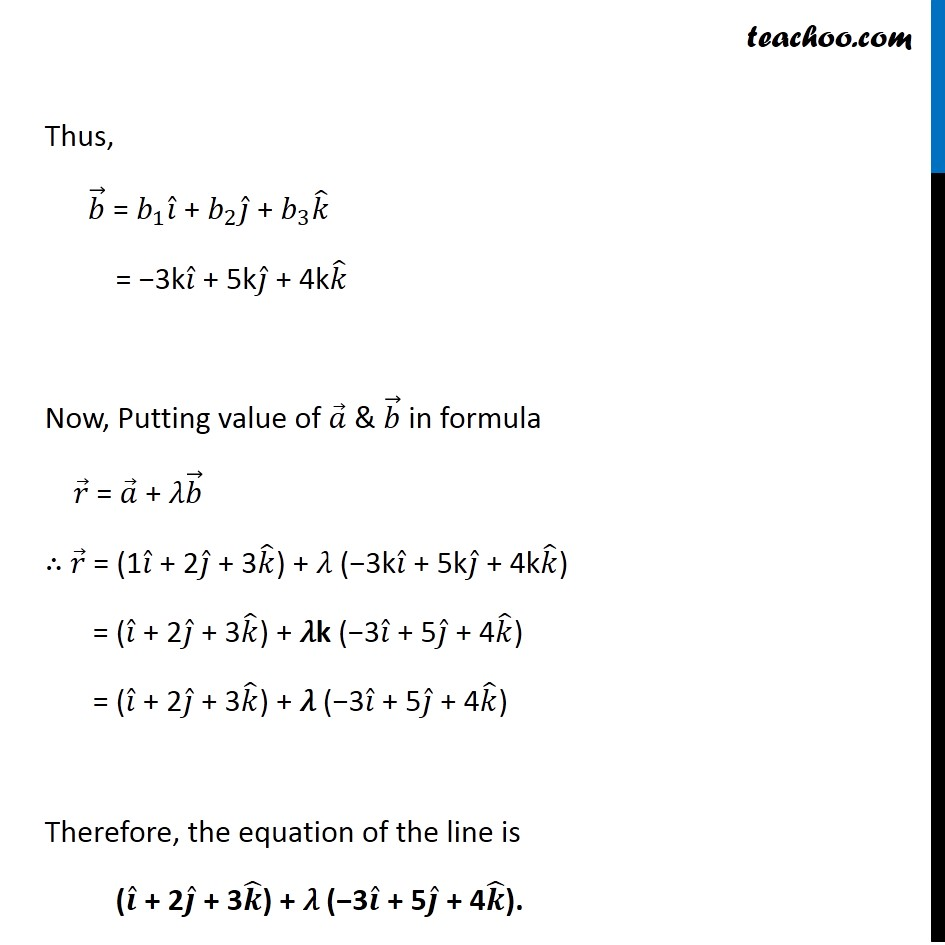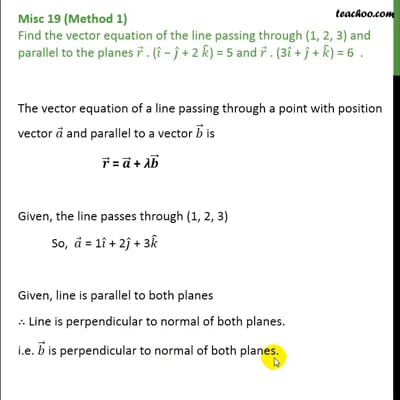This video is only available for Teachoo black users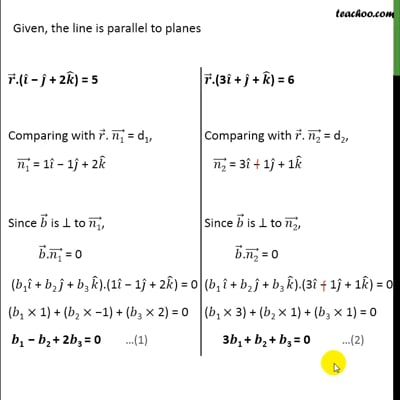This video is only available for Teachoo black users

Solve all your doubts with Teachoo Black (new monthly pack available now!)

### Transcript

Misc 19 (Method 1) Find the vector equation of the line passing through (1, 2, 3) and parallel to the planes 𝑟 ⃗ . (𝑖 ̂ − 𝑗 ̂ + 2 𝑘 ̂) = 5 and 𝑟 ⃗ . (3𝑖 ̂ + 𝑗 ̂ + 𝑘 ̂) = 6 . The vector equation of a line passing through a point with position vector 𝑎 ⃗ and parallel to a vector 𝑏 ⃗ is 𝒓 ⃗ = 𝒂 ⃗ + 𝜆𝒃 ⃗ Given, the line passes through (1, 2, 3) So, 𝑎 ⃗ = 1𝑖 ̂ + 2𝑗 ̂ + 3𝑘 ̂ Given, line is parallel to both planes ∴ Line is perpendicular to normal of both planes. i.e. 𝑏 ⃗ is perpendicular to normal of both planes. The vector equation of a line passing through a point with position vector 𝑎 ⃗ and parallel to a vector 𝑏 ⃗ is 𝒓 ⃗ = 𝒂 ⃗ + 𝜆𝒃 ⃗ Given, the line passes through (1, 2, 3) So, 𝑎 ⃗ = 1𝑖 ̂ + 2𝑗 ̂ + 3𝑘 ̂ Given, line is parallel to both planes ∴ Line is perpendicular to normal of both planes. i.e. 𝑏 ⃗ is perpendicular to normal of both planes. We know that 𝑎 ⃗ × 𝑏 ⃗ is perpendicular to both 𝑎 ⃗ & 𝑏 ⃗ So, 𝑏 ⃗ is cross product of normal of planes 𝑟 ⃗ . (𝑖 ̂ − 𝑗 ̂ + 2 𝑘 ̂) = 5 and 𝑟 ⃗ . (3𝑖 ̂ + 𝑗 ̂ + 𝑘 ̂) = 6 Required normal = |■8(𝑖 ̂&𝑗 ̂&𝑘 ̂@1&−1&2@3&1&1)| = 𝑖 ̂ (–1(1) – 1(2)) – 𝑗 ̂ (1(1) – 3(2)) + 𝑘 ̂(1(1) – 3(–1)) = 𝑖 ̂ (–1 – 2) – 𝑗 ̂ (1 – 6) + 𝑘 ̂(1 + 3) = –3𝑖 ̂ + 5𝑗 ̂ + 4𝑘 ̂ Thus, 𝑏 ⃗ = −3𝑖 ̂ + 5𝑗 ̂ + 4𝑘 ̂ Now, Putting value of 𝑎 ⃗ & 𝑏 ⃗ in formula 𝑟 ⃗ = 𝑎 ⃗ + 𝜆𝑏 ⃗ = (𝒊 ̂ + 2𝒋 ̂ + 3𝒌 ̂) + 𝜆 (−3𝒊 ̂ + 5𝒋 ̂ + 4𝒌 ̂) Now, Putting value of 𝑎 ⃗ & 𝑏 ⃗ in formula 𝑟 ⃗ = 𝑎 ⃗ + 𝜆𝑏 ⃗ = (𝒊 ̂ + 2𝒋 ̂ + 3𝒌 ̂) + 𝜆 (−3𝒊 ̂ + 5𝒋 ̂ + 4𝒌 ̂) Misc 19 (Method 2) Find the vector equation of the line passing through (1, 2, 3) and parallel to the planes 𝑟 ⃗ . (𝑖 ̂ − 𝑗 ̂ + 2 𝑘 ̂) = 5 and 𝑟 ⃗ . (3𝑖 ̂ + 𝑗 ̂ + 𝑘 ̂) = 6 . The vector equation of a line passing through a point with position vector 𝑎 ⃗ and parallel to a vector 𝑏 ⃗ is 𝒓 ⃗ = 𝒂 ⃗ + 𝜆𝒃 ⃗ Given, the line passes through (1, 2, 3) So, 𝑎 ⃗ = 1𝑖 ̂ + 2𝑗 ̂ + 3𝑘 ̂ Let 𝑏 ⃗ = 𝑏_1 𝑖 ̂ + 𝑏_2 𝑗 ̂ + 𝑏_3 𝑘 ̂ A line parallel to a plane is perpendicular to the normal of the plane. And two lines 𝑝 ⃗ and 𝑞 ⃗ are perpendicular if 𝑝 ⃗.𝑞 ⃗ = 0 Given, the line is parallel to planes 𝒓 ⃗.(𝒊 ̂ − 𝒋 ̂ + 2𝒌 ̂) = 5 Comparing with 𝑟 ⃗. (𝑛1) ⃗ = d1, (𝑛1) ⃗ = 1𝑖 ̂ − 1𝑗 ̂ + 2𝑘 ̂ Since 𝑏 ⃗ is ⊥ to (𝑛1) ⃗, 𝑏 ⃗.(𝑛1) ⃗ = 0 (𝑏1𝑖 ̂ + 𝑏2 𝑗 ̂ + 𝑏3 𝑘 ̂).(1𝑖 ̂ − 1𝑗 ̂ + 2𝑘 ̂) = 0 (𝑏"1"× 1) + (𝑏"2"× −1) + (𝑏3 × 2) = 0 𝒃1 − 𝒃2 + 2𝒃3 = 0 𝒓 ⃗.(3𝒊 ̂ + 𝒋 ̂ + 𝒌 ̂) = 6 Comparing with 𝑟 ⃗. (𝑛2) ⃗ = d2, (𝑛2) ⃗ = 3𝑖 ̂ + 1𝑗 ̂ + 1𝑘 ̂ Since 𝑏 ⃗ is ⊥ to (𝑛2) ⃗, 𝑏 ⃗.(𝑛2) ⃗ = 0 (𝑏1 𝑖 ̂ + 𝑏2 𝑗 ̂ + 𝑏3 𝑘 ̂).(3𝑖 ̂ + 1𝑗 ̂ + 1𝑘 ̂) = 0 (𝑏1 × 3) + (𝑏2 × 1) + (𝑏3 × 1) = 0 3𝒃1 + 𝒃2 + 𝒃3 = 0 So, our equations are 𝑏1 − 𝑏2 + 2𝑏3 = 0 3𝑏1 + 𝑏2 + 𝑏3 = 0 Thus, 𝑏 ⃗ = 𝑏_1 𝑖 ̂ + 𝑏_2 𝑗 ̂ + 𝑏_3 𝑘 ̂ = −3k𝑖 ̂ + 5k𝑗 ̂ + 4k𝑘 ̂ Now, Putting value of 𝑎 ⃗ & 𝑏 ⃗ in formula 𝑟 ⃗ = 𝑎 ⃗ + 𝜆𝑏 ⃗ ∴ 𝑟 ⃗ = (1𝑖 ̂ + 2𝑗 ̂ + 3𝑘 ̂) + 𝜆 (−3k𝑖 ̂ + 5k𝑗 ̂ + 4k𝑘 ̂) = (𝑖 ̂ + 2𝑗 ̂ + 3𝑘 ̂) + 𝜆k (−3𝑖 ̂ + 5𝑗 ̂ + 4𝑘 ̂) = (𝑖 ̂ + 2𝑗 ̂ + 3𝑘 ̂) + 𝜆 (−3𝑖 ̂ + 5𝑗 ̂ + 4𝑘 ̂) Therefore, the equation of the line is (𝒊 ̂ + 2𝒋 ̂ + 3𝒌 ̂) + 𝜆 (−3𝒊 ̂ + 5𝒋 ̂ + 4𝒌 ̂).